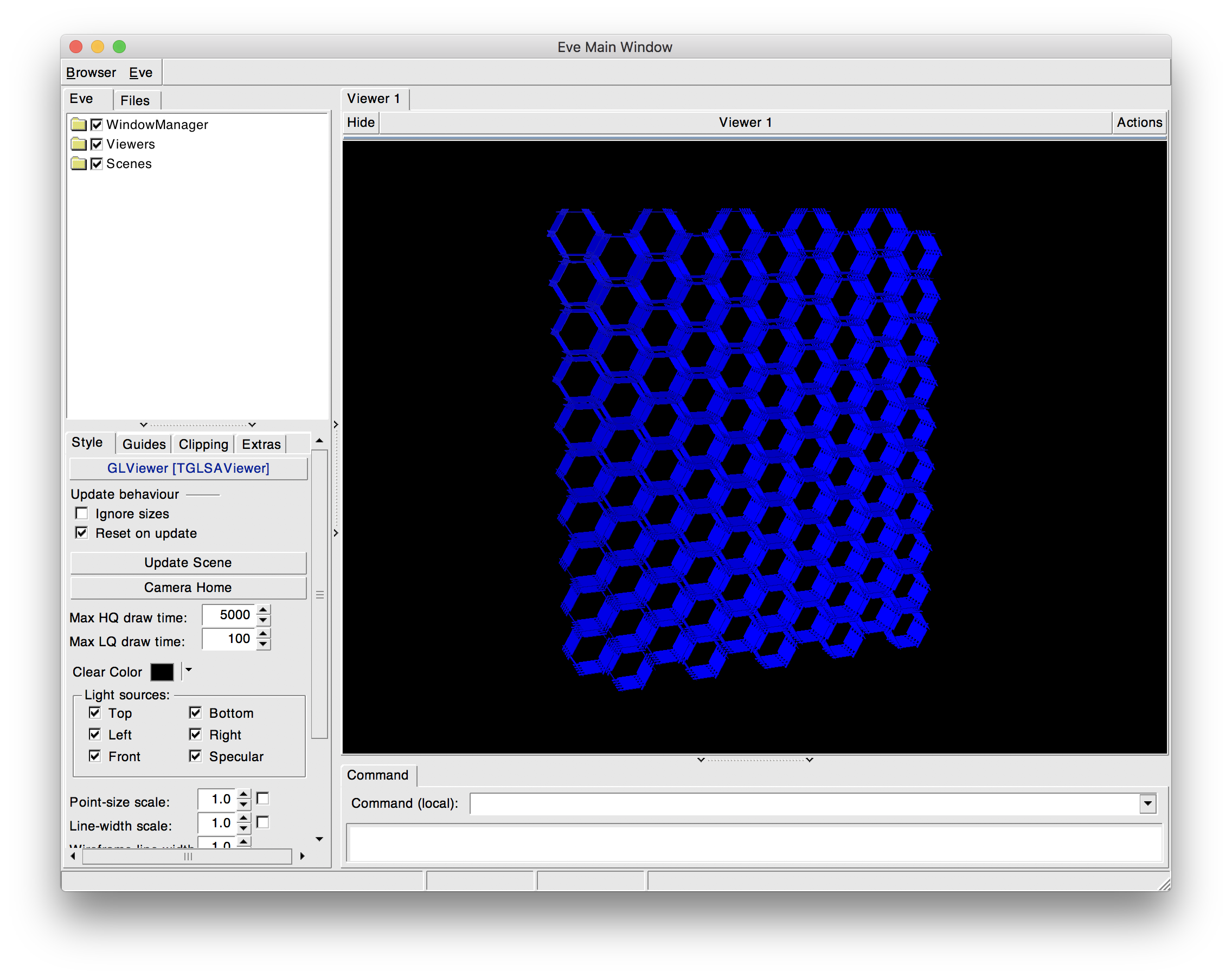ROOT   Reference Guide
Searching...
No Matches
assembly.C File Reference

## Detailed Description

Geometry detector assembly example.

Modified to save the assembly as shape-extract.

1. Run root assembly.C This will produce assembly.root containing the extract.
2. Display the assembly as: root show_extract.C("assembly.root")void assembly()
{
//--- Definition of a simple geometry
TGeoManager *geom = new TGeoManager("Assemblies",
"Geometry using assemblies");
Int_t i;
//--- define some materials
TGeoMaterial *matVacuum = new TGeoMaterial("Vacuum", 0,0,0);
TGeoMaterial *matAl = new TGeoMaterial("Al", 26.98,13,2.7);
// //--- define some media
TGeoMedium *Vacuum = new TGeoMedium("Vacuum",1, matVacuum);
TGeoMedium *Al = new TGeoMedium("Aluminium",2, matAl);
//--- make the top container volume
TGeoVolume *top = geom->MakeBox("TOP", Vacuum, 1000., 1000., 100.);
geom->SetTopVolume(top);
// Make the elementary assembly of the whole structure
TGeoVolume *tplate = new TGeoVolumeAssembly("TOOTHPLATE");
Int_t ntooth = 5;
Double_t xplate = 25;
Double_t yplate = 50;
Double_t xtooth = 10;
Double_t ytooth = 0.5*yplate/ntooth;
Double_t dshift = 2.*xplate + xtooth;
Double_t xt,yt;
TGeoVolume *plate = geom->MakeBox("PLATE", Al, xplate,yplate,1);
TGeoVolume *tooth = geom->MakeBox("TOOTH", Al, xtooth,ytooth,1);
for (i=0; i<ntooth; i++) {
xt = xplate+xtooth;
yt = -yplate + (4*i+1)*ytooth;
xt = -xplate-xtooth;
yt = -yplate + (4*i+3)*ytooth;
}
TGeoRotation *rot1 = new TGeoRotation();
rot1->RotateX(90);
// Make a hexagone cell out of 6 tooth plates. These can zip together
// without generating overlaps (they are self-contained)
TGeoVolume *cell = new TGeoVolumeAssembly("CELL");
for (i=0; i<6; i++) {
Double_t phi = 60.*i;
rot = new TGeoRotation(*rot1);
rot->RotateZ(phi);
}
// Make a row as an assembly of cells, then combine rows in a honeycomb
// structure. This again works without any need to define rows as
// "overlapping"
TGeoVolume *row = new TGeoVolumeAssembly("ROW");
Int_t ncells = 5;
for (i=0; i<ncells; i++) {
Double_t ycell = (2*i+1)*(dshift+10);
}
Double_t dyrow = dshift+10.;
Int_t nrows = 5;
for (i=0; i<nrows; i++) {
Double_t xrow = 0.5*(2*i+1)*dxrow;
Double_t yrow = 0.5*dyrow;
if ((i%2)==0) yrow = -yrow;
}
//--- close the geometry
geom->CloseGeometry();
geom->SetVisLevel(4);
geom->SetVisOption(0);
top->Draw();
}
int Int_t
Definition RtypesCore.h:45
double Double_t
Definition RtypesCore.h:59
@ kBlue
Definition Rtypes.h:66
Class describing rotation + translation.
Definition TGeoMatrix.h:292
The manager class for any TGeo geometry.
Definition TGeoManager.h:45
void SetVisOption(Int_t option=0)
set drawing mode :
void SetVisLevel(Int_t level=3)
set default level down to which visualization is performed
void CloseGeometry(Option_t *option="d")
Closing geometry implies checking the geometry validity, fixing shapes with negative parameters (run-...
TGeoVolume * MakeBox(const char *name, TGeoMedium *medium, Double_t dx, Double_t dy, Double_t dz)
Make in one step a volume pointing to a box shape with given medium.
void SetTopVolume(TGeoVolume *vol)
Set the top volume and corresponding node as starting point of the geometry.
Base class describing materials.
Media are used to store properties related to tracking and which are useful only when using geometry ...
Definition TGeoMedium.h:24
Class describing rotations.
Definition TGeoMatrix.h:175
virtual void RotateX(Double_t angle)
Rotate about X axis of the master frame with angle expressed in degrees.
virtual void RotateZ(Double_t angle)
Rotate about Z axis of the master frame with angle expressed in degrees.
Class describing translations.
Definition TGeoMatrix.h:122
Volume assemblies.
Definition TGeoVolume.h:305
TGeoVolume, TGeoVolumeMulti, TGeoVolumeAssembly are the volume classes.
Definition TGeoVolume.h:49
virtual TGeoNode * AddNode(TGeoVolume *vol, Int_t copy_no, TGeoMatrix *mat=0, Option_t *option="")
Add a TGeoNode to the list of nodes.
virtual void Draw(Option_t *option="")
draw top volume according to option
virtual void SetLineColor(Color_t lcolor)
Set the line color.